StatLect

Test statistic

In a test of hypothesis, the test statistic is a function of the sample data used to decide whether or not to reject the null hypothesis. If the test statistic falls within a critical region, fixed ex-ante, then the null hypothesis is rejected. Otherwise it is not rejected.The test statistic is a random variable

These are the main things to remember about the test statistic:

• it is a single number that summarizes the sample data used to conduct the test of hypothesis;

• before being observed, the sample data is regarded as random; therefore, the test statistic, which depends on random data, is a random variable;

• we need to be able to derive its probability distribution under the null hypothesis (exactly or approximately); otherwise, we won't be able to carry out our test.

How the test statistic is used

The test statistic is used to decide whether to reject or not to reject the null hypothesis, as follows:

1. the set of values it can take (its support) is divided in two parts, called acceptance region and critical region;

2. the sample data is used to calculate the realization of the test statistic;

3. if the realization belongs to the critical region, then the null hypothesis is rejected; otherwise, if the realization belongs to the acceptance region, the null is not rejected.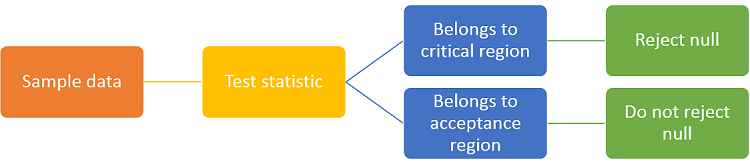How its support is divided into the critical and acceptance regions

In order to decide how to divide the support of the test statistic, we need to:

1. choose the sizeof the test, that is, the probability of incorrectly rejecting the null hypothesis;

2. derive the probability distribution of the test statistic under the null hypothesis;

3. find one or more intervals of values (collectively called the critical region) such that, under the null, the probability that the test statistic belongs to these intervals is equal to the desired size.

The acceptance region is the complement of the critical region. In other words, it is the set of all values that belong to the support of the test statistic but do not belong to the critical region.

Note that the size is usually a small number (such as 5% or 1%) because we want to keep the probability of incorrectly rejecting the null as small as possible. As a consequence, the critical region is usually made up of intervals of values that have small probability and lie in the tails of the probability distribution of the test statistic.

The intuition is that if the null hypothesis is true, then it is an unlikely event that the realization of the test statistic will fall in the critical region. When such an unlikely event happens, then we reject the null hypothesis.

Examples

Some examples of widely used test statistics follow.

In order to fully understand these examples, the reader should be familiar with the concept of critical value.

Example 1 - t statistic

Suppose we observeindependent drawsfrom a normal distribution having unknown meanand unknown variance.

Suppose we want to test the null hypothesis that the mean is equal to a specific value: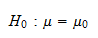A test statistic, called t-statistic, can be constructed with the sample data:whereis the sample mean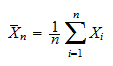andis the adjusted sample variance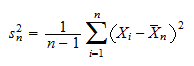It can be proved (see Hypothesis testing about the mean) that the t-statistic has a standard Student's t distribution with-degrees of freedom.

If our test is two-tailed, then the critical region is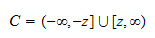and the critical valuesatisfieswhereis the desired size of the test andis the cumulative distribution function of the Student's t distribution withdegrees of freedom evaluated at. The null hypothesis thatis rejected if the test statistic falls within the critical region, that is, ifExample 2 - Chi-square statistic

As in the previous example, suppose we observeindependent drawsfrom a normal distribution having unknown meanand unknown variance.

In this example, we want to test the null hypothesis that the variance is equal to a specific value: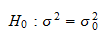The following test statistic, called Chi-square statistic, is employed:whereis the adjusted sample varianceandis the sample meanIt can be demonstrated (see Hypothesis testing about the variance) that the Chi-square statistic has a Chi-square distribution with-degrees of freedom.

If our test is right-tailed, then the critical region isand the critical valuesatisfies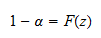whereis the size of the test andis the cumulative distribution function of the Chi-square distribution withdegrees of freedom. The null hypothesis thatis rejected if the realization of the test statistic belongs to the critical region, that is, ifMore examples

The following table contains references to lectures where you can find more examples of test statistics.

Name of the test statistic Distribution of the statistic Lecture where the statistic is introduced
z-statistic Normal distribution Hypothesis testing about the mean
Wald statistic Chi-square distribution Wald test
LR statistic Chi-square distribution Likelihood ratio test
Score statistic Chi-square distribution Score test

How to derive a test statistic

After reading the examples above, you might wonder how the test statistics were derived. How do we know how to obtain a test statistic that can be used to test a given null hypothesis? Unfortunaltely, there is no general rule. As a matter of fact, it often happens that there are several different test statistics we can use to test a given null hypothesis. For example, when conducting a test on a vector of parameters estimated by maximum likelihood, we can choose any one of the three classical statistics: the Wald statistic, the likelihood ratio statistic or the score statistic.

In practice, what we usually do is to frame our null hypothesis in such a way that we can use a known test statistic (one that has already been proposed and whose properties have been studied). So, for instance, we could try to frame our null as an hypothesis about the mean of a distribution (and use a z- or a t-statistic) or as a restriction on a vector of parameters to be estimated by maximum likelihood (and use a Wald, score or likelihood ratio statistic). A part of a statistician's skill set is to know many test statistics and when they can be used. Of course, if you are a skilled statistician, you can build your own test statistic, tailored to the hypothesis you are testing. But how this can be accomplished is beyond the scope of this introduction.

More details

Go to the lecture entitled Hypothesis testing for a rigorous definition of the concept of test statistic.